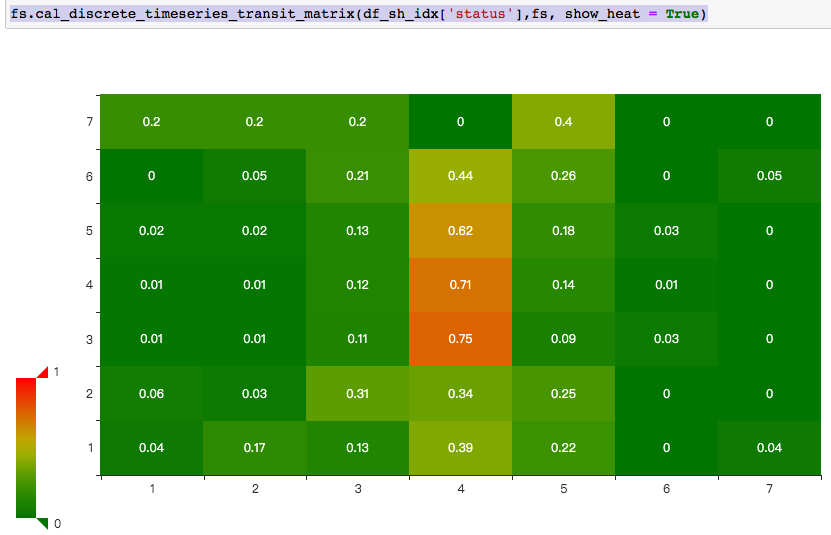内容

1 读取数据

获取所有股票列表

# 股票列表
#查询当前所有正常上市交易的股票列表
stocks_df = pro.stock_basic(exchange='', list_status='L', fields='ts_code,symbol,name,area,industry,list_date')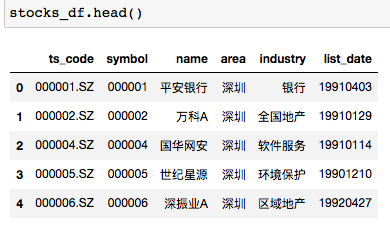获取所有指数列表

# 指数列表
index_df  = pro.index_basic(market='SSE')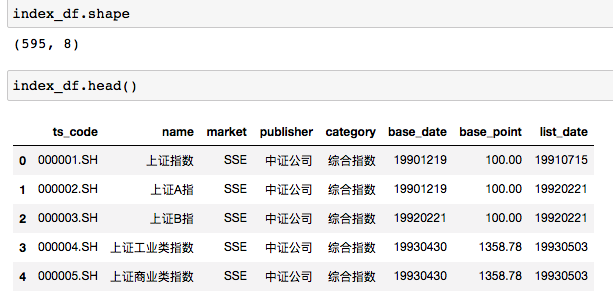2 获取股票数据

# 光明
df_gm = pro.daily(ts_code='600597.SH', start_date='20100701', end_date='20220718')
df_gm['status'] = (df_gm['pct_chg']/100).apply(fs.stock_change_status)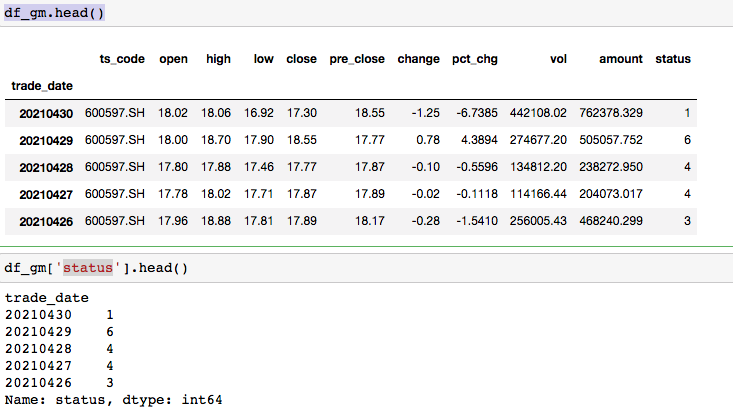# 对一个都是离散值（状态）的时间序列，计算转移矩阵
def cal_discrete_timeseries_transit_matrix(some_s ,fs, show_heat = False,missing_pct = 0.0001):
# 1 获取时间状态序列的差分
tem_df = fs.s_trans_pair(some_s)
# 2 差分序列转矩阵
trans_mat = fs.s_trans_pair2mat(tem_df)
# 3 计算转移概率矩阵
trans_mat_pct = fs.s_trans_mat2pct(trans_mat)
trans_mat_pct = trans_mat_pct.fillna(missing_pct)
if show_heat:
cols_name_list,rows_name_list, data_list = fs.make_pe_heatmap_para(trans_mat_pct)
return fs.pe_heat_map(cols_name_list,rows_name_list, data_list)
else:
return trans_mat_pct

fs.cal_discrete_timeseries_transit_matrix(df_gm['status'],fs, show_heat = True)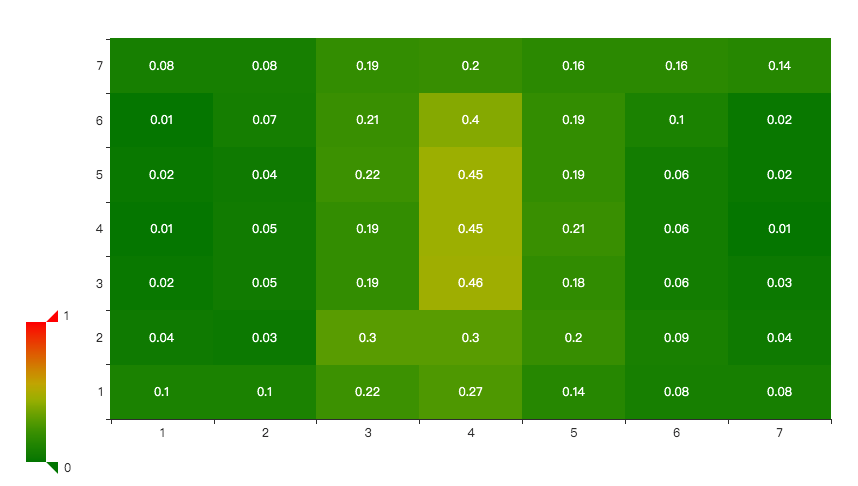2021.4.30的状态是1， 所以下一个个交易日上涨(5,6,7)的概率是30%，继续下跌的概率是24%。从概率转移矩阵上看，处于1（超级跌），2（大跌）之后行情处于更不确定的状态。以4（振荡）为中心，概率分布更加扁平。而3，4，5状态之后的变化更加确定，表现为集中在中间。在不确定的情况下，应该见好就收。

fs.cal_discrete_timeseries_transit_matrix(df_sh_idx['status'],fs, show_heat = True)